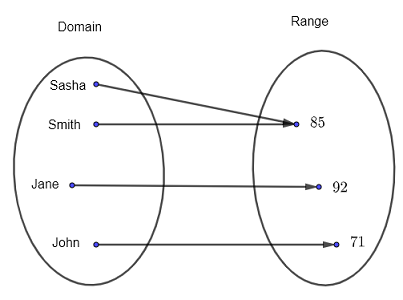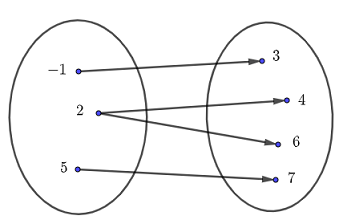# Relations in Mathematics

  

The definition of a relation in mathematics, along with its domain and range, is presented with examples, questions and their solutions.

## Definition of a Relation in Mathematics

A relation is a correspondence between two sets called the domain and the range .

Example 1
The relation $R_1$ between a group of four students $\{ \; \text{Sasha}\; , \; \text{Smith} \; , \; \text{Jane} \; , \; \text{John} \; \}$ and their exam scores $\{ \; 85 \; , \; 92 \; , \; 71 \; \}$ is shown in the diagram below.Fig.1 - Relation $R_1$ Between Students and Their Scores
The above
relation $R_1$ may be represented as a set of ordered pairs as follows: $\quad R_1 = \{ \; (\text{Sasha} \; , \; 85) \; , \; (\text{Smith} \; , \; 85) \; , \; (\text{Jane} \; , \; 92) \; , \; (\text{John} \; , \; 71) \; \}$
The first element in each ordered pair is an element of the domain and the second element in the pair is the corresponding element of the range.
The
domain $D$ of the relation $R_1$ is a set given by: $\quad D = \{ \; \text{Sasha}\; , \; \text{Smith} \; , \; \text{Jane} \; , \; \text{John} \; \}$
The
range $R$ of the relation $R_1$ is a set given by: $\quad R = \{ \; 85 \; , \; 92 \; , \; 71 \; \}$

Example 2
The relation $R_2$ between the time at which matches are to be played $\{ 10 \; \text{am} \; , \; 1 \; \text{pm} \; , \; 4 \; \text{pm} \}$ and the pairs of teams $\{ \text{A vs B} \; , \; \text{C vs D} \; , \; \text{E vs F} \; , \; \text{G vs H} \}$ to play at that time is shown below.Fig.2 - Relation $R_2$ Time and Matches
The above
relation $R_2$ may be represented as a set of ordered pairs as follows: $\quad R_2 = \{ \; (10 \; \text{am} \; , \; \text{A vs B}) \; , \; (1 \; \text{pm} \; , \; \text{C vs D}) \; , \; (4 \; \text{pm} \; , \; \text{E vs F}) \; , \; (4 \; \text{pm} \; , \; \text{G vs H}) \; \}$
Note that the first element in the ordered pair is an element of the domain and the second element in the pair is the corresponding element of the range.
The
domain $D$ of the relation $R_2$ is a set given by: $\quad D = \{ \; 10 \; \text{am} \; , \; 1 \; \text{pm} \; , \; 4 \; \text{pm} \; \}$
The
range $R$ of the relation $R_2$ is a set given by: $\quad R = \{ \; \text{A vs B} \; , \; \text{C vs D} \; , \; \text{E vs F} \; , \; \text{G vs H} \; \}$

## Representations of Relations

Using the same relation, we describe some of the most important representations of relations in mathematics.

### Relations Represented by Venn Diagrams

Relations use a set for the domain and a set for the range as shown in figure 3 and arrows from each element in $D$ to the corresponding element in the range $R$.

### Relations Represented as a Set of Ordered Pairs

A relation may also be represented as a set of ordered pairs as shown below. The first element in an ordered pair is an element in the domain $D$ and the second element is the corresponding element in the range $R$. $R_3 = \{ \; (2,3) \; , \; (4,1) \; , \; (6,4) \; , \; (7,2) \; , \; (7,6) \; \}$

### Relations Represented by a Table

A relation may be represented by a table with two rows (or two columns). A row $x$ with elements in the domain $D$ and a row $y$ with the corresponding elements in the range $R$ as shown below.

 $x$ $2$ $4$ $6$ $7$ $7$ $y$ $3$ $1$ $4$ $2$ $6$

### Relations Represented by Graphs

Use a system of rectangular coordinates where each ordered pair is represented by a point $(x,y)$ where $x$ is an element in the domain $D$ and $y$ is the corresponding element in the range $R$.

### Relations Represented by Equations

The equation $y = 3 x + 1$ represents a relation between $x$ and $y$. Given values of the variable $x$ in the domain $D$ of the relation, we can find the corresponding values of $y$ in the range $R$.
For example, for $x = \color{red}{2}$, we calculate the corresponding value of $y$ by substituting $x$ by $\color{red}{2}$ in the given equation as follows:
$y = 3(\color{red}{2}) + 1$
We simplify to obtain:
$y = 7$
If we are given several values of $x$, it is better to use a table as follows:

 $x$ $\color{red}{-3}$ $\color{red} 0$ $\color{red} 5$ $\color{red} 8$ $y$ $3(\color{red}{-3}) + 1 = - 8$ $3(\color{red}{0}) + 1 = 1$ $3(\color{red}{5}) + 1 = 16$ $3(\color{red}{8}) + 1 = 25$

Example 3
Given the relation $R_4= \{ \; (-1 , 3) \; , \; (2 , 4) \; , \; (5,7) \; , \; (2 , 6) \; \}$ as a set of ordered pairs,
a) find its domain and range,
and represent it as
b) a graph
c) table
d) a Venn diagram.

Solution to Example 3
a)
The relation $R_4$ is given as a set of ordered pairs: $\{ \; (\color{red}{-1} , \color{blue}{3}) \; , \; (\color{red}{2} , \color{blue}{4}) \; , \; (\color{red}{5},\color{blue}{7}) \; , \; (\color{red}{2} , \color{blue}{6}) \; \}$
The domain $D$ is the set of all values of the first element in the ordered pairs.
$\color{red}{D = \{ \; -1 , 2 , 5 \; \}}$
The domain $R$ is the set of all values of the second element in the ordered pairs.
$\color{blue}{R = \{ \; 3 , 4 , 6 , 7 \; \}}$
b)
Relation $R_4$ represented by a graph is shown in figure 5 below where each point correspond to an ordered pair in the given relation.Fig.4 - Relation $R_4$ Represented by a graph for Example 3
c)
The given relation $R_4$ represented by a table is shown below
 $x$ $-1$ $2$ $2$ $5$ $y$ $3$ $4$ $6$ $7$

d)
Relation $R_4$ represented by a Venn diagram is shown in figure 5 below.Fig.5 - Relation $R_4$ Represented by a Venn diagram for Example 3

## Questions

### Part A

Relation $R_5$ is given by its graph in figure 6 below.
a) Represent relation $R_5$ a) a set of ordered pairs, b) as a table, b) , c) using Venn diagram.
b) Find its domain and range.Fig.6 - Relation Represented by a Graph for Questions Part A

### Part B

Relation $R_6$ is given by a table below.

 $x$ $-2$ $-2$ $-2$ $0$ $1$ $3$ $y$ $3$ $4$ $5$ $4$ $0$ $0$

a) Find its domain and range.
Represent it by b) a set of ordered pairs, c) a Venn diagram.

### Part C

Given answers in ordered pairs representation.
a) Give an example of two different relations with the same domain.
b) Give an example of two different relations with the same range.
c) Give an example of two different relations with the same domain and range.

### Part D

A relation is defined by the equation $y + 4 x = 2$ where $x$ is a variable in the domain of the relation taking the values: $\{ -1 , 0 , 1 , 2 \}$.
a) Use a table to find the $y$ values corresponding to the given $x$ values.
b) Represent the relation using a graph.

## Solutions to the Above Questions

### Part A

a)
Relation $R_5$ as a set of ordered pairs is given by: $\quad R_5 = \{ \; (-2,2) \; , \; (1,1) \; , \; (2,1) \; , \; (2,5) \; , \; (4,2) \; , \; (4,3) \; , \;(4,4) \; \}$
b)
Relation $R_5$ as table
 $x$ $-2$ $1$ $2$ $2$ $4$ $4$ $4$ $y$ $2$ $1$ $1$ $5$ $2$ $3$ $4$
c)
Relation $R_5$ using Venn diagramFig.7 - Relation $R_5$ Using Venn diagram for Question in Part A

### Part B

a) The domain $D$ is the set of all first terms in the ordered pair: $D = \{ -2 \; , \; 0 \; , \; 1 \; , \; 3 \}$
The range $R$ is the set of all second terms in the ordered pair: $R = \{ 3 \; , \; 4 \; , \; 5 \; , \; 0 \}$
b) Relation $R_6$ as an ordered pair: $\{ \; (-2,3) \; , \; (-2,4) \; , \; (-2,5) \; , \; (0,4) \; , \; (1,0) \; , \; (3,0) \; \}$
c) Relation $R_6$ represented by Venn diagramFig.7 - Relation $R_6$ Using Venn diagram for Question in Part B

### Part C

a) The relations $\; (-3,3) \; , \; (-2,4) \; , \; (0,5) \; , \; (4,5) \;$ and $\; (-3,3) \; , \; (-2,8) \; , \; (0,5) \; , \; (4,0) \;$ have the same domain but are different.
b) The relations $\; (0,3) \; , \; (-2,5) \; , \; (6,5) \; , \; (7,5) \;$ and $\; (-3,3) \; , \; (-2,5) \; , \; (0,5) \; , \; (9,5) \;$ have the same range but are different.
c) The relations $\; (-1,0) \; , \; (0,-5) \; , \; (4,5) \; , \; (7,8) \;$ and $\; (-1,0) \; , \; (-1,-5) \; , \; (0,-5) \; , \; (4,5) \; , \; (7,8) \;$ have the same domain and range but are different.

### Part D

The relation is defined by the equation: $y + 4 x = 2$ which may be written as $y = - 4x + 2$
a) The variable $x$ takes the values: $\{ -1 , 0 , 1 , 2 \}$, hence the table of values of $x$ and corresponding $y$ calculated using the given equation as follows:

 $x$ $-1$ $0$ $1$ $2$ $y$ $y = - 4(-1) + 2 = 6$ $y = - 4(0) + 2 = 2$ $y = - 4(1) + 2 = -2$ $y = - 4(2) + 2 = -6$

b) The graph corresponding to the relation defined by the equation $y + 4 x = 2$ and with domain $\{ -1 , 0 , 1 , 2 \}$ is shown below.Fig.7 - Relation $y + 4 x = 2$ Represented by a Graph for Question in Part D